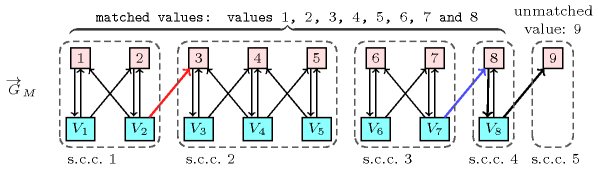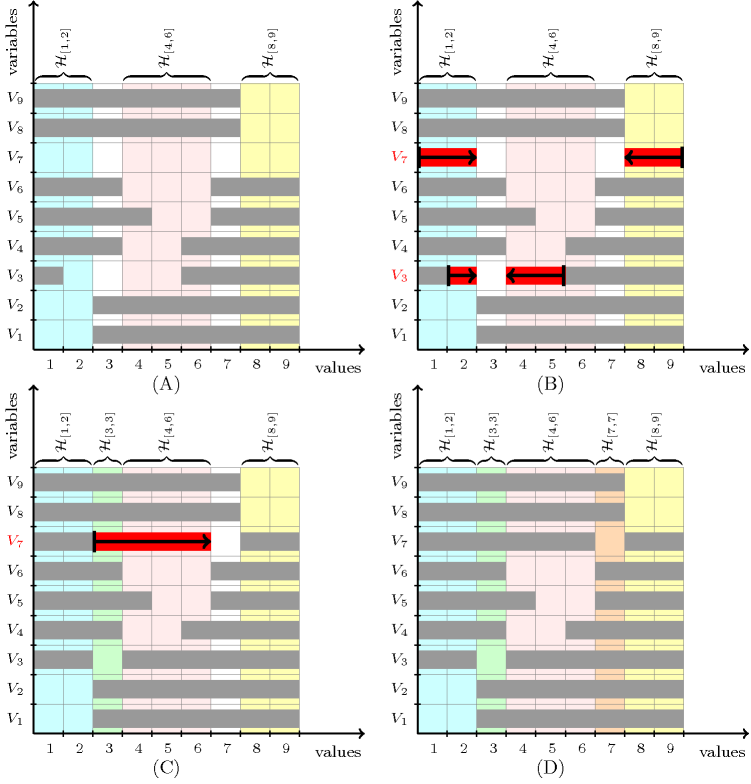### 2.1.3. Filtering view

Once we know that a constraint for which not all variables are fixed yet is potentially feasible, the next question is to identify variable-value pairs of the form $\left(\mathrm{𝑣𝑎𝑟},\mathrm{𝑣𝑎𝑙}\right)$ such that, if value $\mathrm{𝑣𝑎𝑙}$ is assigned to variable $\mathrm{𝑣𝑎𝑟}$, the constraint has no solution. Since removing such values reduces a priori the search space, filtering is strongly supported by the implicit motto of constraint programming that the more you prune the better. Assuming that you already have a necessary and sufficient condition that can be evaluated in polynomial time for checking whether a constraint has at least one solution or not, you can directly reuse it for checking whether a value can be assigned or not to a variable. Since the number of variable-value pairs to check may be quadratic with respect to the total number of variables and values one usually prefers developing a dedicated filtering algorithm that is less costly than checking the feasibility condition on each variable-value pair.

For the $\mathrm{𝚊𝚕𝚕𝚍𝚒𝚏𝚏𝚎𝚛𝚎𝚗𝚝}$ constraint a first filtering algorithm [Regin94] is based on a characterisation of the edges of the variable-value graph that belong to a maximum matching but not to all. A matching on a graph is a set of edges of the graph such that no two edges have a vertex in common; it is maximum if its number of edges is maximum. We first introduce a digraph ${\stackrel{\to }{G}}_{M}$ that is associated with a matching $M$ that matches all variables of the $\mathrm{𝚊𝚕𝚕𝚍𝚒𝚏𝚏𝚎𝚛𝚎𝚗𝚝}$ constraint. The vertices of ${\stackrel{\to }{G}}_{M}$ are defined as the variables and the values that can be assigned to the variables of the $\mathrm{𝚊𝚕𝚕𝚍𝚒𝚏𝚏𝚎𝚛𝚎𝚗𝚝}$ constraint. To each value $\mathrm{𝑣𝑎𝑙}$ that can be assigned to a variable $\mathrm{𝑣𝑎𝑟}$ corresponds an arc of ${\stackrel{\to }{G}}_{M}$ from $\mathrm{𝑣𝑎𝑟}$ to $\mathrm{𝑣𝑎𝑙}$. Finally, if value $\mathrm{𝑣𝑎𝑙}$ is matched to variable $\mathrm{𝑣𝑎𝑟}$ in the matching $M$ we add the reverse arc from $\mathrm{𝑣𝑎𝑙}$ to $\mathrm{𝑣𝑎𝑟}$ to the arcs of ${\stackrel{\to }{G}}_{M}$. Now a variable $\mathrm{𝑣𝑎𝑟}$ can be assigned a value $\mathrm{𝑣𝑎𝑙}$ if and only if $\mathrm{𝑣𝑎𝑟}$ and $\mathrm{𝑣𝑎𝑙}$ belong to the same strongly connected component of ${\stackrel{\to }{G}}_{M}$ or if there is a path consisting of distinct vertices and arcs of ${\stackrel{\to }{G}}_{M}$ that start with $\left(\mathrm{𝑣𝑎𝑟},\mathrm{𝑣𝑎𝑙}\right)$ and ends up in an unmatched value with respect to matching $M$, see [Thiel04].

##### Figure 2.1.1. Illustration of the filtering for $\mathrm{𝚊𝚕𝚕𝚍𝚒𝚏𝚏𝚎𝚛𝚎𝚗𝚝}$$\left(〈{V}_{1},{V}_{2},{V}_{3},{V}_{4},{V}_{5},{V}_{6},{V}_{7},{V}_{8}〉\right)$ with ${V}_{1}\in \left[1,2\right]$, ${V}_{2}\in \left[1,3\right]$, ${V}_{3}\in \left[3,4\right]$, ${V}_{4}\in \left[3,5\right]$, ${V}_{5}\in \left[4,5\right]$, ${V}_{6}\in \left[6,7\right]$, ${V}_{7}\in \left[6,8\right]$, ${V}_{8}\in \left[8,9\right]$ with respect to the maximum matching $M$ defined by ${V}_{i}=i$ ($1\le i\le 8$) and the corresponding digraph and its five strongly connected components s.c.c. $k$ ($1\le k\le 5$): 8 can be assigned to ${V}_{7}$ (blue arc) since the path $\left({V}_{7}8{V}_{8}9\right)$ ends up in an unmatched value, but 3 cannot be assigned to ${V}_{2}$ (red arc) since 3 and ${V}_{2}$ do not belong to the same strongly connected component and since there is no path from ${V}_{2}$ to the unique unmatched value 9.Another filtering algorithm for $\mathrm{𝚊𝚕𝚕𝚍𝚒𝚏𝚏𝚎𝚛𝚎𝚗𝚝}$ based on Hall intervals just focuses on adjusting the minimum and maximum values of the variables. Given a set of domain variables, a Hall interval is an interval of values $\left[\mathrm{𝑙𝑜𝑤},\mathrm{𝑢𝑝}\right]$ denoted by ${ℋ}_{\left[\mathrm{𝑙𝑜𝑤},\mathrm{𝑢𝑝}\right]}$ such that there are $\mathrm{𝑢𝑝}-\mathrm{𝑙𝑜𝑤}+1$ variables whose domains are contained in ${ℋ}_{\left[\mathrm{𝑙𝑜𝑤},\mathrm{𝑢𝑝}\right]}$. A minimal Hall interval ${ℋ}_{\left[\mathrm{𝑙𝑜𝑤},\mathrm{𝑢𝑝}\right]}$ is a Hall interval that does not contain any Hall interval ${ℋ}_{\left[{\mathrm{𝑙𝑜𝑤}}^{\text{'}},{\mathrm{𝑢𝑝}}^{\text{'}}\right]}$ such that either $\mathrm{𝑙𝑜𝑤}={\mathrm{𝑙𝑜𝑤}}^{\text{'}}$ or $\mathrm{𝑢𝑝}={\mathrm{𝑢𝑝}}^{\text{'}}$. Given a Hall interval $ℋ$ and a variable $V$ whose domain is not included in $ℋ$ but intersects $ℋ$, the idea is to adjust the minimum (respectively maximum) value of variable $V$ to the smallest (respectively largest) value that does not belong to $ℋ$. Figure 2.1.2 illustrates this idea on the constraint $\mathrm{𝚊𝚕𝚕𝚍𝚒𝚏𝚏𝚎𝚛𝚎𝚗𝚝}$$\left(〈{V}_{1},{V}_{2},{V}_{3},{V}_{4},{V}_{5},{V}_{6},{V}_{7},{V}_{8},{V}_{9}〉\right)$ with ${V}_{1}\in \left[1,2\right]$, ${V}_{2}\in \left[1,2\right]$, ${V}_{3}\in \left[2,5\right]$, ${V}_{4}\in \left[4,5\right]$, ${V}_{5}\in \left[5,6\right]$, ${V}_{6}\in \left[4,6\right]$, ${V}_{7}\in \left[1,9\right]$, ${V}_{8}\in \left[8,9\right]$, ${V}_{9}\in \left[8,9\right]$.

• Part (A) of Figure 2.1.2 shows in light blue, in light pink and in light yellow the three minimal Hall intervals associated with the initial domains of variables ${V}_{1},{V}_{2},\cdots ,{V}_{9}$.

• ${ℋ}_{\left[1,2\right]}$ corresponds to interval $\left[1,2\right]$, which contains the domains of ${V}_{1}$ and ${V}_{2}$.

• ${ℋ}_{\left[4,6\right]}$ corresponds to interval $\left[4,6\right]$, which contains the domains of ${V}_{4}$, ${V}_{5}$ and ${V}_{6}$.

• ${ℋ}_{\left[8,9\right]}$ corresponds to interval $\left[8,9\right]$, which contains the domains of ${V}_{8}$ and ${V}_{9}$.

• Part (B) of Figure 2.1.2 shows the first propagation step with respect to the Hall intervals ${ℋ}_{\left[1,2\right]}$, ${ℋ}_{\left[4,6\right]}$ and ${ℋ}_{\left[8,9\right]}$. First note that variables ${V}_{3}$ and ${V}_{7}$ are the only variables whose domain is not included in a Hall interval. Consequently ${V}_{3}$ and ${V}_{7}$ are candidates for adjusting their minimum or maximum domain values.

• Since the minimum value of ${V}_{3}$, that is i.e. value 2, belongs to the Hall interval ${ℋ}_{\left[1,2\right]}$ we adjust the minimum of ${V}_{3}$ to the smallest value that does not yet belong to any Hall interval, i.e. value 3.

• Since the maximum value of ${V}_{3}$, that is i.e. value 5, belongs to the Hall interval ${ℋ}_{\left[4,6\right]}$ we adjust the maximum value of ${V}_{3}$ to the largest value that does not yet belong to any Hall interval, i.e. value 3.

• Since the minimum value of ${V}_{7}$, that is i.e. value 1, belongs to the Hall interval ${ℋ}_{\left[1,2\right]}$ we adjust the minimum of ${V}_{7}$ to the smallest value that does not yet belong to any Hall interval, i.e. value 3.

• Since the maximum value of ${V}_{7}$, that is i.e. value 9, belongs to the Hall interval ${ℋ}_{\left[8,9\right]}$ we adjust the maximum of ${V}_{7}$ to the largest value that does not yet belong to any Hall interval, that is i.e. value 7.

• Part (C) of Figure 2.1.2 shows the second propagation step with respect to the new Hall interval ${ℋ}_{\left[3,3\right]}$. Now ${V}_{7}$ is the only variable whose domain is not included in a Hall interval so it is a candidate for adjusting its minimum or maximum domain value.

• Since the minimum value of ${V}_{7}$, i.e. value 3, belongs to the new Hall interval ${ℋ}_{\left[3,3\right]}$ we adjust the minimum of ${V}_{7}$ to the smallest value that does not yet belong to any Hall interval, i.e. value 7.

• Finally, part (D) of Figure 2.1.2 shows all the minimal Hall intervals after reaching the fix point of the filtering, where ${ℋ}_{\left[7,7\right]}$ is a new minimal Hall interval.

##### Figure 2.1.2. Steps (A), (B), (C) and (D) for filtering the minimum and maximum values wrt Hall intervals ${ℋ}_{\left[\mathrm{𝑙𝑜𝑤},\mathrm{𝑢𝑝}\right]}$ for $\mathrm{𝚊𝚕𝚕𝚍𝚒𝚏𝚏𝚎𝚛𝚎𝚗𝚝}$$\left(〈{V}_{1},{V}_{2},{V}_{3},{V}_{4},{V}_{5},{V}_{6},{V}_{7},{V}_{8},{V}_{9}〉\right)$ with ${V}_{1}\in \left[1,2\right]$, ${V}_{2}\in \left[1,2\right]$, ${V}_{3}\in \left[2,5\right]$, ${V}_{4}\in \left[4,5\right]$, ${V}_{5}\in \left[5,6\right]$, ${V}_{6}\in \left[4,6\right]$, ${V}_{7}\in \left[1,9\right]$, ${V}_{8}\in \left[8,9\right]$, ${V}_{9}\in \left[8,9\right]$. Each horizontal grey strip corresponds to a set of consecutive values that do not belong to the domain of a variable, while each horizontal red strip represents an adjustment of the minimum or the maximum value of the domain of a variable.